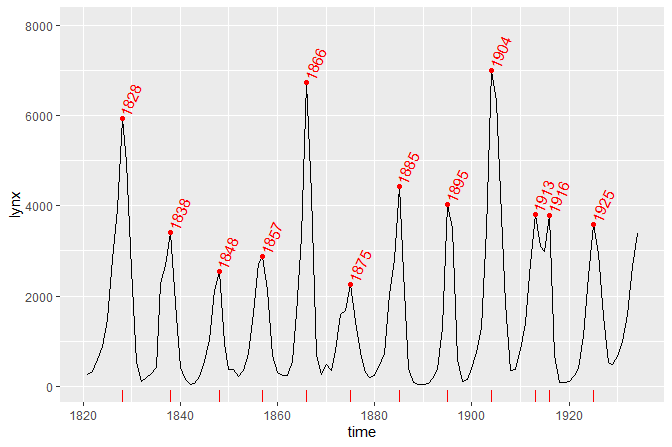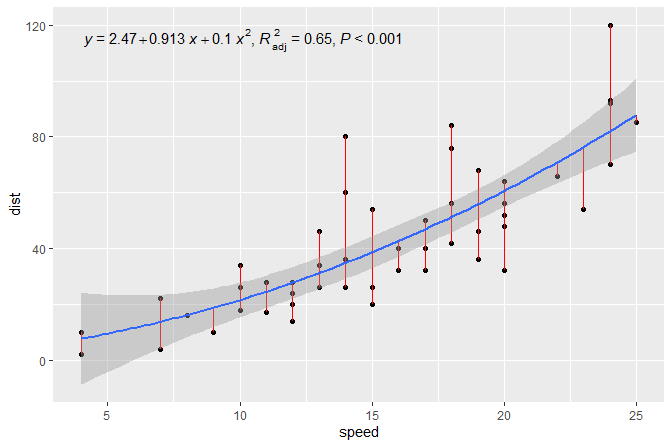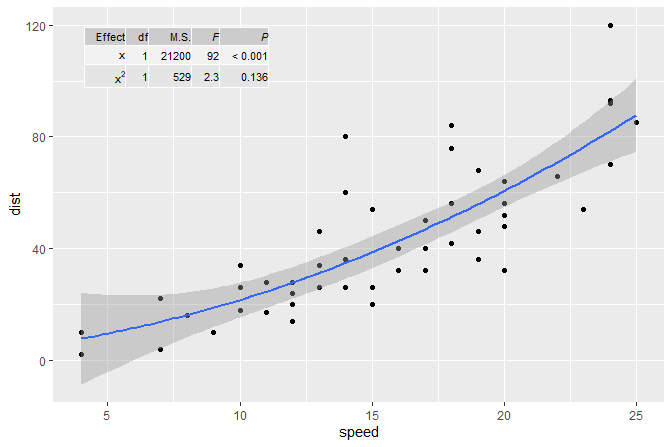ggpmiscPurpose

Package ‘ggpmisc’ (Miscellaneous Extensions to ‘ggplot2’) is a set of extensions to R package ‘ggplot2’ (>= 3.0.0) with emphasis on annotations and highlighting related to fitted models and data summaries. Data summaries shown as text, tables or equations are implemented. The location of such summaries in the plotting area is usually set independently of the `x` and `y` scales. The same applies to plot insets and logos and other arbitrary graphical elements. The “natural” coordinates to use in such cases are expressed in ‘grid’ “npc” units in the range [0..1].

Geometries

Geometries `geom_table()`, `geom_plot()` and `geom_grob()` make it possible to add inset tables, inset plots, and arbitrary ‘grid’ graphical objects as layers to a ggplot using native coordinates for `x` and `y`. Geometries `geom_text_npc()`, `geom_label_npc()`, `geom_table_npc()`, `geom_plot_npc()` and `geom_grob_npc()` are versions that interpret `x` and `y` values as if expressed in “npc” units.

Statistics

Statistic `stat_fmt_tb()` helps with the formatting of tabbles to be plotted with `geom_table()`. Statistics `stat_peaks()` and `stat_valleys()` can be used to highlight and/or label maxima and minima in a plot. `ggplot` constructors for time series objects. The provided `ggplot.ts()` and `ggplot.xts()` use `try_tibble()` which is also exported and accepts objects of additional classes as input. Statistics that help with reporting the resulst of model fits are `stat_poly_eq()`, `stat_fit_residuals()`, `stat_fit_deviations()`, `stat_fit_broom()`, `stat_fit_broom_tb()`. A summary statistic using special grouping for quadrants `stat_quadrant_counts()` can be used to automate labelling with the number of observations. `stat_apply_panel()` and `stat_apply_group()` can be useful for applying arbitrary summary functions. Two statistics, `stat_dens2d_filter()` and `stat_dens2d_label()`, implement tagging or selective labelling of observations based on the local 2D density of observations. These two stats are designed to work well together with `geom_text_repel()` and `geom_label_repel()` from package ‘ggrepel’.

ggplot methods

Being `ggplot()` defined as a generic method in ‘ggplot2’ makes it possible to define specializations, and we provide two for time series stored in objects of classes `ts` and `xts` which automatically convert these objects into tibbles and set the as default the aesthetic mappings for `x` and `y`. A companion fucntion `try_tibble()` is also exported.

MIGRATED

Functions for the manipulation of layers in ggplot objects and statistics and geometries that echo their data input to the R console, earlier included in this package are now in package ‘gginnards’.

Examples

``library(ggpmisc)``

In the first example we plot a time series using the specialized version of `ggplot()` that converts the time series into a tibble and maps the `x` and `y` aesthetics automatically. We also highlight and label the peaks using `stat_peaks`.

``````ggplot(lynx, as.numeric = FALSE) + geom_line() +
stat_peaks(colour = "red") +
stat_peaks(geom = "text", colour = "red", angle = 66,
hjust = -0.1, x.label.fmt = "%Y") +
stat_peaks(geom = "rug", colour = "red", sides = "b") +
expand_limits(y = 8000)``````In the second example we add the equation for a fitted polynomial plus the adjusted coefficient of determination to a plot showing the observations plus the fitted curve, deviations and confidence band. We use `stat_poly_eq()`.

``````formula <- y ~ x + I(x^2)
ggplot(cars, aes(speed, dist)) +
geom_point() +
stat_fit_deviations(method = "lm", formula = formula, colour = "red") +
geom_smooth(method = "lm", formula = formula) +
stat_poly_eq(aes(label =  paste(..eq.label.., ..adj.rr.label.., sep = "~~~~")),
formula = formula, parse = TRUE)``````The same figure as in the second example but this time annotated with the ANOVA table for the model fit. We use `stat_fit_tb()` which can be used to add ANOVA or summary tables.

``````formula <- y ~ x + I(x^2)
ggplot(cars, aes(speed, dist)) +
geom_point() +
geom_smooth(method = "lm", formula = formula) +
stat_fit_tb(method = "lm",
method.args = list(formula = formula),
tb.type = "fit.anova",
tb.vars = c(Effect = "term",
"df",
"M.S." = "meansq",
"italic(F)" = "statistic",
"italic(P)" = "p.value"),
label.y.npc = "top", label.x.npc = "left",
size = 2.5,
parse = TRUE)
#> Warning in seq.default(along = x): partial argument match of 'along' to
#> 'along.with'``````A plot with an inset plot.

``````library(tibble)
p <- ggplot(mtcars, aes(factor(cyl), mpg, colour = factor(cyl))) +
stat_boxplot() +
labs(y = NULL) +
theme_bw(9) + theme(legend.position = "none")
df <- tibble(x = 0.01, y = 0.015, plot = list(p))
ggplot(mtcars, aes(wt, mpg, colour = factor(cyl))) +
geom_point() +
geom_plot_npc(data = df, mapping = aes(npcx = x, npcy = y, label = plot),
vjust = 0, hjust = 0) +
expand_limits(y = 0, x = 0)``````Installation

Installation of the most recent stable version from CRAN:

``install.packages("ggspectra")``

Installation of the current unstable version from Bitbucket:

``````# install.packages("devtools")
devtools::install_bitbucket("aphalo/ggspectra")``````

Documentation

HTML documentation is available at (https://docs.r4photobiology.info/ggpmisc/), including a User Guide.

News on updates to the different packages of the ‘r4photobiology’ suite are regularly posted at (https://www.r4photobiology.info/).

Contributing

Please report bugs and request new features at (https://bitbucket.org/aphalo/ggpmisc/issues). Pull requests are welcome at (https://bitbucket.org/aphalo/ggpmisc).

Please note that this project is released with a Contributor Code of Conduct. By participating in this project you agree to abide by its terms.

Citation

If you use this package to produce scientific or commercial publications, please cite according to:

``````citation("ggpmisc")
#>
#> To cite ggpmisc in publications, please use:
#>
#>   Pedro J. Aphalo. (2016) Learn R ...as you learnt your mother
#>   tongue. Leanpub, Helsinki.
#>
#> A BibTeX entry for LaTeX users is
#>
#>   @Book{,
#>     author = {Pedro J. Aphalo},
#>     title = {Learn R ...as you learnt your mother tongue},
#>     publisher = {Leanpub},
#>     year = {2016},
#>     url = {https://leanpub.com/learnr},
#>   }``````